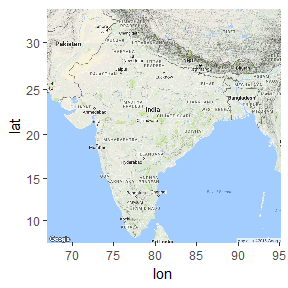# Animated Map of PM2.5 concentrations in India

#### 2016-09-14

The goal of this vignette is to show how to use the gganimate package (see https://github.com/dgrtwo/gganimate) for making an animated map of air PM2.5 concentrations in India. Obviously this could be adapted to other subsets of OpenAQ data.

library("ggmap")
library("ggplot2")
library("ropenaq")
library("dplyr")
library("tidyr")

# First, get the data and calculate daily average

We only query locations for which we have geographical information and we only want positive concentrations. Since there are many measurements we need to loop over several pages.

measurementsIndia <- NULL
for (page in 1:7){
print(page)
measurementsIndia <- rbind(measurementsIndia,
aq_measurements(country = "IN",
has_geo = TRUE,
page = page,
parameter = "pm25",
limit = 1000,
date_from = "2015-09-01",
value_from = 0))}

# Calculate daily averages

dailyIndia <- measurementsIndia %>%
group_by(day = as.Date(dateLocal),
location) %>%
summarize(value = mean(value)) %>%
mutate(location = as.factor(location))

dailyIndia <- complete(dailyIndia, day, location, fill = list(value = NA))

tableGeo <- unique(select(measurementsIndia, location,
longitude, latitude))

dailyIndia <- dailyIndia %>% left_join(tableGeo)
#> Joining, by = "location"
#> Warning in left_join_impl(x, y, by$x, by$y, suffix$x, suffix$y): joining
#> character vector and factor, coercing into character vector

# Open map for India

indiaMap <- get_map(location = c(65,
6,
97,
36))
#> Warning: bounding box given to google - spatial extent only approximate.
#> converting bounding box to center/zoom specification. (experimental)
#> Map from URL : http://maps.googleapis.com/maps/api/staticmap?center=21,81&zoom=5&size=640x640&scale=2&maptype=terrain&language=en-EN&sensor=false
ggmap(indiaMap)# Make the animated map

You’ll need to have installed both gganimate (see https://github.com/dgrtwo/gganimate) and animation package (from CRAN), and also have the ffmpeg software (https://www.ffmpeg.org/)

You’ll find the resulting video in the vignettes folder.

library("gganimate")
library("animation")
minConc <- min(dailyIndia$value) maxConc <- max(dailyIndia$value)
plotMap <- ggmap(indiaMap)+ theme_bw()+
geom_point(data = dailyIndia, aes(x=longitude,
y=latitude,
frame=day,
colour = value),
size=8)+
low="yellow",
high="red") +
theme(axis.line=element_blank(),axis.text.x=element_blank(),
axis.text.y=element_blank(),axis.ticks=element_blank(),
axis.title.x=element_blank(),
text = element_text(size=20),
axis.title.y=element_blank(),
panel.background=element_blank(),panel.border=element_blank(),panel.grid.major=element_blank(),
panel.grid.minor=element_blank(),plot.background=element_blank())+
ggtitle("PM 2.5 concentration") +
theme(plot.title = element_text(lineheight=1, face="bold"))

ani.options(interval = 0.25, ani.width = 800, ani.height = 800)
gg_animate(plotMap, "map.mp4")